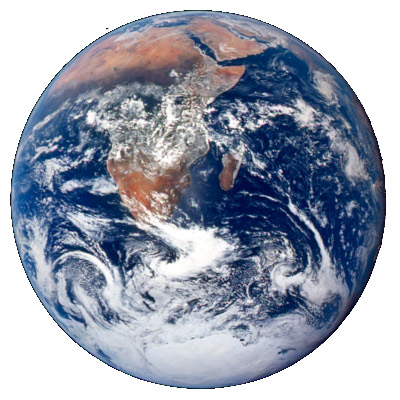# A tale of three citiesPick three cities and form a spherical triangle by connecting each pair of cities with the shortest arc between them. How might you find the area of this triangle?

For this post, I’ll assume the earth is perfectly spherical. (Taking into account that the earth is slightly oblate makes the problem much more complicated. Maybe a post for another time.)

## Spherical excess

If you draw a triangle on the plane, the interior angles add up to 180°. But if you draw a triangle on a sphere, the interior angles add up to more than 180°. The amount by which the sum of the interior angles exceeds 180° is called the spherical excess. It turns out that the area of a spherical triangle is proportional to its spherical excess.

For example, if a spherical triangle is small relative to the radius of the sphere, it’s nearly a Euclidean triangle, and the spherical excess is negligible. But consider a much bigger spherical triangle, one with a vertex at the north pole and vertices at two points 90° apart on the equator. This triangle has three right angles, so the sum of the interior angles is 270° and the spherical excess is 90°.

## Relating spherical excess and area

If we measure spherical excess E in radians, then the area of a spherical triangle is

A = ER²

where R is the radius of the sphere. This remarkable theorem was discovered by Thomas Harriot in 1603.

Let’s go back to the example above of a triangle running from the north pole to two points 90° apart on the equator. This triangle takes up 1/8 of the earth’s surface, and area of the earth is 4πR², and so the triangle has area πR²/2. In this case the spherical excess E was π/2, and so we could have come to the same result by multiplying E by R².

## Computing area

We can find the area of a spherical triangle by measuring the angle of arcs between pairs of points. We’ll compute area assuming the sphere has radius 1. (Otherwise temporarily assume radius 1 and then multiply by R² when we’re done.)

The previous post explains how to find a parameterization for the great circle connecting points on a sphere. We can take derivatives to find tangent vectors where the great circles intersect, and compute the angle between these tangents to find the interior angles of our triangles.

The previous post showed that if the central angle between two vectors A and B is θ then

cos(t) A + sin(t) (cot θ A – csc θ B)

parameterizes a great circle including A and B. This curve passes through A at time t = 0 with velocity

cot θ A – csc θ B

and so this gives us a tangent vector at A in the direction of B. Repeat the exercise for a great circle between A and C. Then the cosine of the angle of intersection is the two normalized tangent vectors. We can thus obtain all the interior angles, and from there we can compute the spherical excess and thus the area of the spherical triangle.

## 2 thoughts on “A tale of three cities”

1. Yves Caudano

There is also a very nice formula that relates the solid angle to the three normalised vectors a, b, c through the triple product and the three scalar products of the vector pairs:
tan E/2 = (a x b).c / (1 + a.b + b.c + c.a)

(see “On the measure of solid angles”, F. Eriksson, Math. Mag. 63 (1990) 184–187)